Courses

# Context Free Grammars (CFG) Computer Science Engineering (CSE) Notes | EduRev

## Computer Science Engineering (CSE) : Context Free Grammars (CFG) Computer Science Engineering (CSE) Notes | EduRev

The document Context Free Grammars (CFG) Computer Science Engineering (CSE) Notes | EduRev is a part of the Computer Science Engineering (CSE) Course Theory of Computation.
All you need of Computer Science Engineering (CSE) at this link: Computer Science Engineering (CSE)

Context Free Grammars and Languages

As we learned in first module, according to Chomsky classification, Type- 2 languages are also called context free languages. They are generated using context free grammars. They are recognised using push down automata.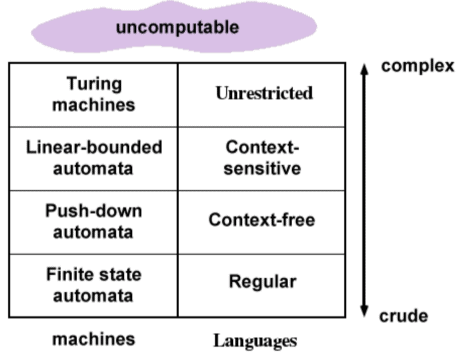Also regular languages are a subset of context free languages.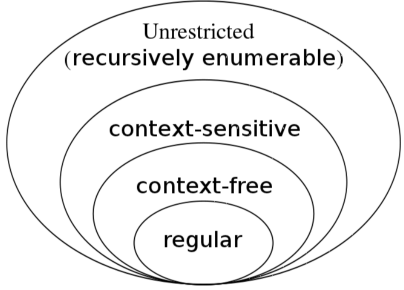Part I. Context Free Grammars (CFG)
Context free grammar, G is defined as,
G = (V,∑, P, S)
where
V is a set of non-terminals,
∑ is a set of terminals,
S is the start symbol,
P is a set of productions.

A grammar, G is context free, if productions are of the form, α → β
where α is a single non terminal.

Every regular grammar is context free.

Example 1:
An example for context free grammar is shown below:
S → ASA|BSB|a|b
A → a
B → b
where S is the start symbol.

From the definition of CFG,
G = {S, A, B}
P = {a, b}
P = {S → ASA|BSB|a|b, A → a, B → b}
S = S

The production,
S → ASA|BSB|a|b
can also be written as,
S → ASA
S → BSB
S → a
S → b

Example 2:
The following is an example for context free grammar.
Stmt → id = Expr;
Stmt → if (Expr) Stmt
Stmt → if (Expr) Stmt else Stmt
Stmt → while (Expr) Stmt
Stmt → do Stmt while (Expr);
Stmt→ { Stmts }

Stmts → Stmts Stmt| ε
where Stmts is the start symbol.

From the definition of CFG,
G = {Stmts, stmt, Expr}
∑ = {id, =, ; ,(,), if, else, while, do}
P is the set of productions given above
S = Stmts

Note that above CFG corresponds to some statements in C programming language.

1 Language of Context Free Grammar (Context Free Language)
A language, L of G is a set of strings that can be derived from G.

Example 1:
Consider the following context free grammar,
S → aA|bB
A → x
B → y
The language corresponding to the above CFG is {ax,by}

A string w belongs to a context free language (CFL), L, if it can be derived from the CFG associated with L.
That is,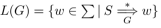There are two approaches to check whether a string belongs to a CFL. They are, Derivations, and Reductions.

Derivations
It is a mechanism to check whether a string w belongs to a context free language (CFL).
Example 1:
Consider the following CFG,
S → aSb | ab
where S is the start symbol.
Check whether the string aabb belongs to the CFL corresponding to above CFG.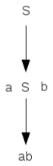Above diagram is called a derivation tree (parse tree).
Here, by performing two derivations, we got a2b2. So a2bbelongs to the above CFL.

Example 2:
Consider the following CFG,
S → aSa | bSb |c
where S is the start symbol.
Check whether the string abbcbba belongs to the language corresponding to the above grammar.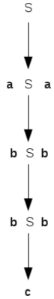From the above derivation tree, the string abbcbba is derived using the given CFG. So the string abbcbba belongs to the above language.

Example 3:
Consider the CFG,
S → aAS|a|SS
A → SbA|ba
where S is the start symbol.
Check whether the string aabaa can be derived using the above grammar.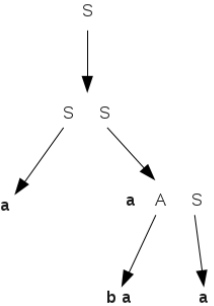Example 4:
Consider the CFG,
S → aAS|a
A → SbA|SS|ba
where S is the start symbol.
Check whether the string aabbaa belongs to this language.
Following is the derivation tree: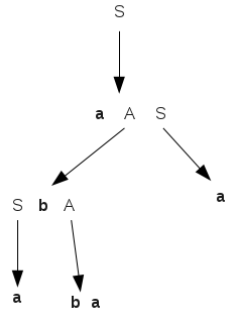Thus the above string aabbaa or a2b2a2 belongs to the above CFL.

Reductions
This approach can also be used to check whether a string belongs to a context free language. Here, the string w is takenand an inverted tree is made. this is done by performing a number of reductions starting from the given string using the productions of the grammar.

Example 1:
Consider the grammar,
S → aAS|a|SS
A → SbA|ba
where S is the start symbol.
Check whether the string aabaa belongs to the CFL associated with the above grammar.
The reduction tree is shown below: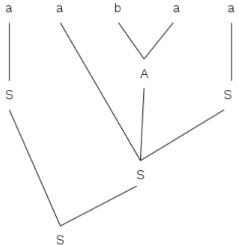Thus by performing a number of reductions, we finally reached the start symbol. So the string aabaa belongs to the above CFL.

2 CFG corresponding to a CFL
Example 1:
Design a CFG for the language,
L = {anbn| n ≥ 0}
At n=0, ε is a valid string for the above language. The production for this is,
S → ε
Every string is of length 2n. The strings are ab, abab, aaabbb, ......... etc..
The corresponding production is,
S → aSb.
Hence the grammar is,
S → aSb | ε
where S is the start symbol.

Example 2:
Design a CFG for the language,
L = {anb2n| n ≥ 0}
At n=0, ε is a valid string for the above language. The production for this is,
S → ε
It can be seen that there are double the number of b’s as a’s. The production is,
S → aSbb
So the grammar is,
S → aSbb | ε
where S is the start symbol.

Example 3:
Design a CFG for the language, L over {0,1} to generate all possible strings of even length.

Consider number 0 as even. The string of length 0 is ε. The corresponding production is,
S → ε
An even length is of length 2n contains one or more repetitions of 00, 01, 10 or 11. The corresponding production is,
S → 00S|01S|10S|11S

Thus the grammar is,
S → 00S|01S|10S|11S|ε

Example 4:
Design a CFG for the language, L over {0,1} to generate all the strings having alternate sequence of 0 and 1.

The minimum string length in L is 2. The strings are 01 or 10.
If a string begins with 01, then it should follow a repetition of 01 and terminate with either 01 or 0.
If a string begins with 10, then it should follow a repetition of 10 and terminate with either 10 or 1.
The grammar will be,
S → 01|10|01A|10B
A → 01A|0|01
B → 10B|1|10

Example 5:
Write a CFG for the regular expression,
a*b(a|b)*

On analysing this regular expression, we can see that the strings start with any number of a’s, followed by a ’b’ and may
end with a combination of a’s and b’s.
The CFG can be written as,
S → AbB
A → aA|ε
B → aB|bB|ε

For example, using the above productions

S ⇒ AbB
⇒ aAbB
⇒ aAbaB
⇒ aaAbaB
⇒ aaAbabB
⇒ aabab
aabab is string belonging to the above CFL.

Example 6:
Write a CFG which generates strings of equal number of a’s and b’s.
The CFG is
S → aSbS|bSaS|ε

For example, using the above productions,
S ⇒ bSaS
⇒ bbSaSaS
⇒ bbaSbSaSaS
⇒ bbaSbaSbSaSaS
⇒ bbababaa
Note that above string contains equal number of a’s and b’s.

Example 7:
Design a CFG for the regular expression,
(a|b)*
The CFG is,
S → aS
S → bS
S → ε
That is,
S → aS|bS|ε
where S is the start symbol.

Example 8;
Design a CFG for the language,
L(G) = {wwR|w ∈ {0, 1}* }

The CFG is,
S → 0S0|1S1|ε
where S is the start symbol.
[In the above, wR means reverse of string w.]

For example, using the above productions,
S ⇒ 0S0
⇒ 00S00
⇒ 001S100
⇒ 001100

Example 9:
Design a CFG for the language,
L = {anbm| n 6 ≠ m}

Case 1: For n>m,
The language is
L = {anbm| n > m}

The CFG is,
S→ AS1
S1 → aS1b|ε
A → aA|a

Case 2: For n<m,
The language is
L = {anbm| n < m}

The CFG is,
S→ S2B
S2 → aS2b|ε
B → bB|b

Combining Case 1 and Case 2, we get,
S → S1|S2

Thus the CFG is,
S → S1|S22
S1 → AS1
S1 → aS1b|ε
A → aA|a
S2→ S2B
S2 → aS2b|ε
B → bB|b
where S is the start symbol.

Example 10:
Design a CFG for the language,
L = { (0n1n| n ≥ 0) ∪ (1n0n| n ≥ 0) }

We can write it as,
L = L1 ∪ L2

Case 1:
Consider L1,
L1 = { 0n1n| n ≥ 0 }

The CFG is,
S1 → 0S11|ε

Case 2:
Consider L2,
L2 = { 1n0n| n ≥ 0 }

The CFG is,
S2 → 1S20|ε

Then we have L = L∪ L2
Combining above two cases, we get the CFG as,
S → S1|S2

Thus the complete CFG is,
S → S1|S2
S1 → 0S11|ε
S2 → 1S20|ε
where S is the start symbol.

Example 11:
Design a CFG for the language, L
L = { anb2n| n ≥ 0 }

The CFG is,
S → aSbb|ε

Example 12;
Write a CFG for the language,
L = { a2nbm| n > 0, m ≥ 0 }

From the above we can say that L is the set of strings starting with even number of a’s followed by any number of b’s. There is no ’a’ in the string after first ’b’ is encountered. Also there is no ’b’ before any ’a’ in the string.
The CFG is,
S → aaAB
A → aaA|ε
B → bB|ε
where S is the start symbol.

Example 13:
Write a CFG for the language,
L = {anb2ncm| n, m ≥ 0}

This means strings start with ’a’ or ’c’, but not with a ’b’.
If the string starts with ’a’, then number of a’s must follow b’s, and the number of b’s is twice than number of a’s.
If the string starts with ’c’, it is followed by any number of c’s.
There is no ’a’ after any ’b’ or any ’c’.
There is no ’b’ after any ’c’.
There is no ’b’ or ’c’ after any ’a’.
There is no ’c’ after any ’b’.
Thus the CFG is,
S → AB
A → aAbb|ε
B → cB|ε
where S is the start symbol.

Example 14:
Design a CFG for the language,
L = {anbmc2m| n, m ≥ 0}

The CFG is
S → AB
A → aA|ε
B → bBcc|ε

Example 15:
Design a CFG for the language, L = {wcwR|w ∈ (a, b)*}
The CFG is
S → aSa
S → bSb
S → c
where S is the start symbol.

Offer running on EduRev: Apply code STAYHOME200 to get INR 200 off on our premium plan EduRev Infinity!

## Theory of Computation

18 videos|43 docs|39 tests

,

,

,

,

,

,

,

,

,

,

,

,

,

,

,

,

,

,

,

,

,

;